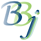# BBjAPI::getFunctionDebugNanoTimes

## Description

This method is used by the BBj Function Debugging subsystem.

## Syntax

Return Value

Method

HashMap<String,Long>

getFunctionDebugNanoTimes()

None.

## Return Value

A map keyed by function name, containing all functions used during this BBjServices run (or since resetFunctionDebug() was called). This returns total execution time for each function, measured in nanoseconds.

## Remarks

For complete details, see BBj Function Debugging.

## Example

Copy
BBjAPI::getFunctionDebugNanoTimes Example
``rem ' BBj Function Debuggingrem ' Enable by adding this setting to BBj.properties:rem ' com.basis.bbj.funcs.debug=trueuse com.basis.bbj.funcs.FunctionMgrprecision 9print "com.basis.bbj.funcs.debug=",print FunctionMgr.DEBUGprint "resetFunctionDebug()"bbjapi().resetFunctionDebug()print "Use some BBj functions...."print cvs(sys,3)," ",revprint date(0:"%hz:%mz:%sz %p")a = sqr(2)b = sqr(100)c = len("The quick brown fox jumps over the lazy dog.")d = len("")print "Report function timing statistics...."counts! = bbjapi().getFunctionDebugCounts()times! = bbjapi().getFunctionDebugNanoTimes()print counts!print times!iter! = counts!.entrySet().iterator()while iter!.hasNext()  entry! = iter!.next()  func\$ = entry!.getKey()  count = entry!.getValue()  nano = times!.get(func\$)  print func\$," was used",count," time(s) for a total of",  print nano/1000000," ms (average",nano/count/1000000," ms)"wendend``

## Sample Run

Copy
BBjAPI::getFunctionDebugNanoTimes Sample Run
``com.basis.bbj.funcs.debug=trueresetFunctionDebug()Use some BBj functions....LEVEL 6 REV 21.0201:36:34 pmReport function timing statistics....{BBJAPI=1, CVS=1, DATE=1, LEN=2, REV=1, SQR=2, SYS=1}{BBJAPI=4821, CVS=18971, DATE=204105, LEN=2742, REV=19530, SQR=89605, SYS=2259}BBJAPI was used 1 time(s) for a total of .004821 ms (average .004821 ms)CVS was used 1 time(s) for a total of .018971 ms (average .018971 ms)DATE was used 1 time(s) for a total of .204105 ms (average .204105 ms)LEN was used 2 time(s) for a total of .002742 ms (average .001371 ms)REV was used 1 time(s) for a total of .01953 ms (average .01953 ms)SQR was used 2 time(s) for a total of .089605 ms (average .0448025 ms)SYS was used 1 time(s) for a total of .002259 ms (average .002259 ms) READY>``